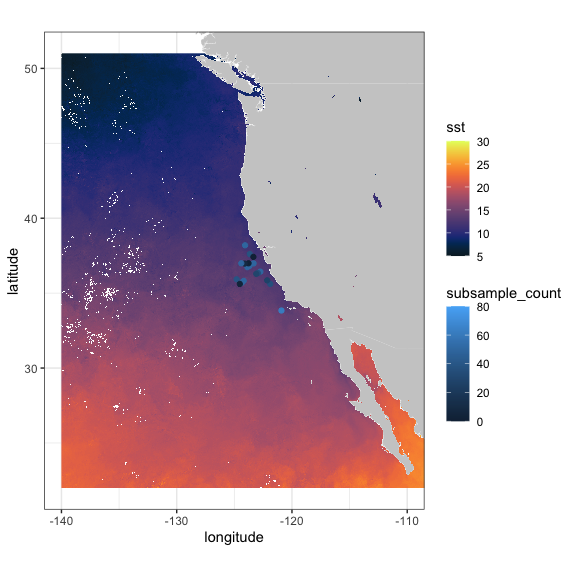# Using rerddap to Access Data from ERDDAP Servers

## Introduction

`rerddap` is a general purpose R client for working with ERDDAP servers. ERDDAP is a web service developed by Bob Simons of NOAA. At the time of this writing, there are over sixty ERDDAP servers (though not all are public facing) providing access to literally petabytes of data and model output relevant to oceanography, meteorology, fisheries and marine mammals, among other areas. ERDDAP is a simple to use, RESTful web service, that allows data to be subsetted and returned in a variety of formats.

This vignette goes over some of the nuts and bolts of using the `rerddap` package, and shows the power of the combination of the `rerddap` package with ERDDAP servers. Some of the examples are taken from the `xtractomatic` package (available from CRAN - https://cran.r-project.org/package=xtractomatic), and some from the `rerddapXtracto` package available on Github (https://github.com/rmendels/rerddapXtracto), but reworked to use `rerddap` directly. Other examples are new to this vignette, and include both gridded and non-gridded datasets from several ERDDAPs.

## Installation

The first step is to install the `rerddap` package, the stable version is available from CRAN:

``install.packages("rerddap")``

or the development version can be installed from GitHub:

``remotes::install_github("ropensci/rerddap")``

``library("rerddap")``

Besides `rerddap` the following libraries are used in this vignette:

``````library("akima")
library("dplyr")
library("ggplot2")
library("mapdata")
library("ncdf4")
library("plot3D")``````

Code chunks are always given with the required libraries so that the chunks are more standalone in nature. Many of the plots use an early version of the `cmocean` colormaps designed by Kristen Thyng (see https://matplotlib.org/cmocean/ and https://github.com/matplotlib/cmocean) translatd from the original Python implementation. However, there is now a `cmocean` package for R. As past scripts may use the built-in color palette it is maintained here, but use of the `cmocean` package is advisable as it is kept up-to-date. In the present version of the `cmocean` package the names have changed, what is “temperature” here is now “thermal”, “chlorophyll” is now “algae”, and “salinity” is now “haline”.

## The main `rerddap` functions

The complete list of rerddap functions can be seen by looking at he `rerddap` package help:

``?rerddap``

and selecting the index of the package. The main functions used here are:

• the list of servers `rerddap` knows about - `server()`
• search an ERDDAP server for terms - `ed_search(query, page = NULL, page_size = NULL, which = "griddap", url = eurl(), ...)`
• search a list of ERDDAP servers for terms - `global_search(query, server_list, which_service)`
• get a list of datasets on an ERDDAP server - `ed_datasets(which = "tabledap", url = eurl())`
• obtain information about a dataset - `info(datasetid, url = eurl(), ...)`
• extract data from a griddap dataset - `griddap(x, ..., fields = "all", stride = 1, fmt = "nc", url = eurl(), store = disk(), read = TRUE, callopts = list())`
• extract data from a tabledap dataset - `tabledap(x, ..., fields = NULL, distinct = FALSE, orderby = NULL, orderbymax = NULL, orderbymin = NULL, orderbyminmax = NULL, units = NULL, url = eurl(), store = disk(), callopts = list())`

Be careful when using the functions `ed_search()`, `ed_datasets()` and `global_search()`. The default ERDDAP has over 9,000 datasets, most of which are grids, so that a list of all the gridded datasets can be quite long. A seemly reasonable search:

``whichSST <- ed_search(query = "SST")``

returns about 1000 responses. The more focused query:

``whichSST <- ed_search(query = "SST MODIS")``

still returns 172 responses. If the simple search doesn’t narrow things enough, look at the advanced search function `ed_search_adv()`.

## Finding the Data You Want

The first way to find a dataset is to browse the builtin web page for a particular ERDDAP server. A list of some of the public available ERDDAP servers can be obtained from the `rerddap` command:

``````servers()
#> # A tibble: 61 × 4
#>    name                                                  short_name url   public
#>    <chr>                                                 <chr>      <chr> <lgl>
#>  1 St. Lawrence Global Observatory - CIOOS | Observatoi… SLGO-OGSL  http… TRUE
#>  2 CoastWatch West Coast Node                            CSWC       http… TRUE
#>  3 ERDDAP at the Asia-Pacific Data-Research Center       APDRC      http… TRUE
#>  4 NOAA's National Centers for Environmental Informatio… NCEI       http… TRUE
#>  5 Biological and Chemical Oceanography Data Management… BCODMO     http… TRUE
#>  6 European Marine Observation and Data Network (EMODne… EMODnet    http… TRUE
#>  7 European Marine Observation and Data Network (EMODne… EMODnet P… http… TRUE
#>  8 Marine Institute - Ireland                            MII        http… TRUE
#>  9 CoastWatch Caribbean/Gulf of Mexico Node              CSCGOM     http… TRUE
#> 10 NOAA IOOS Sensors ERDDAP                              IOOS-Sens… http… TRUE
#> # ℹ 51 more rows``````

The list of ERDDAP servers is based on the list maintained at the Awesome ERDDAP site compiled by the Irish Marine Institute.

The second way to find and obtain the desired data is to use functions in `rerddap`. The basic steps are:

1. Find the dataset on an ERDDAP server (`rerddap::servers()`, `rerddap::ed_search()`, `rerddap::ed_datasets()` ).
2. Get the needed information about the dataset (`rerddap::info()` )
3. Think about what you are going to do.
4. Make the request for the data (`rerddap::griddap()` or `rerddap::tabledap()` ).

We discuss each of these steps in more detail, and then look at some realistic uses of the package.

### Think about what you are going to do.

This may seem to be a strange step in the process, but it is important because many of the datasets are high-resolution, and data requests can get very large, very quickly. As an example, based on a real use case. The MUR SST ( Multi-scale Ultra-high Resolution (MUR) SST Analysis fv04.1, see https://coastwatch.pfeg.noaa.gov/erddap/griddap/jplMURSST41.html ) is a daily, high-quality, high-resolution sea surface temperature product. The user wanted the MUR data for a 2x2-degree box, daily for a year. That seems innocuous enough. Except that MURsst is at a one-hundreth of degree resolution. If we assume just a binary representation of the data, assuming 8-bytes per value, and do the math:

``````100*100*4*8*365
#>  116800000``````

Yes, 116,800,000 bytes or roughly 115MB for that request. Morever the user wanted the data as a .csv file, which usually makes the resulting file 8-10 times larger, so now we are over a 1GB for the request. Even more so, there are four parameters in that dataset, and in `rerddap::griddap()` if “fields” is not specified, all fields are downloaded, therefore the resulting files will be four times as large as given above.

So the gist of this is to think about your request before you make it. Do a little mental math to get a rough estimate of the size of the download. There are times receiving the data as a .csv file is convenient, but make certain the request will not be too large. For larger requests, obtain the data as netCDF files. By default, `rerddap::griddap()` “melts”” the data into a dataframe, so a .csv only provides a small convenience. But for really large downloads, you should select the option in `rerddap::griddap()` to not read in the data, and use instead the `netcdf4` package to read in the data, as this allows for only reading in parts of the data at a time. Below we provide a brief tutorial on reading in data using the `ncdf4` package.

## Some ERDDAP Basics

One of the main advantages of a service such as ERDDAP is that you only need to download the subset of the data you desire, rather than the entire dataset, which is both convenient and essential for large datasets. The underlying data model in ERDDAP is quite simple - everything is either a (multi-dimensional) grid (think R array) or a table (think a simple spreadsheet or data table). Grids are subsetted using the function `griddap()` and tables are subset using the function `tabledap()`.

If you know the datasetID of the data you are after, and are unsure if it is a grid or a table, there are several ways to find out. We will look at two datasets, ‘jplMURSST41’ and ‘siocalcofiHydroCasts’. The first method is to use the `rerddap` function `browse()`

``````browse('jplMURSST41')
browse('siocalcofiHydroCast')``````

which brings up information on the datasets in the browser, in the first case the “data” link is under “griddap”, the second is under “tabledap”.

The other method is to use the `rerddap` function `info`:

``````info('jplMURSST41')
info('siocalcofiHydroCast')``````

Notice the information on ‘jplMURSST41’ lists the dimensions (that is a grid) while that of ‘siocalcofiHydroCasts’ does not (that is a table).

### Subsetting griddap()

Like an R array, ERDDAP grids are subsetted by setting limits on the dimension variables, the difference being that a subset is defined in coordinate space (latitude values, longitude values, time values) rather than array space as is done with R arrays. Thus for ‘jplMURSST41’ if the desired area of the data extract is latitude limits of (22N, 51N), longitude limits of (140W, 105W), and with time limits of (2017-01-01, 2017-01-02) the following would be passed to the function `griddap()`:

``````latitude = c(22., 51.)
longitude = c(-140., -105)
time = c("2017-01-01", "2017-01-02")``````

A full `griddap()` request to retrieve “analysed_sst” with these constraints would be:

``````sstInfo <- info('jplMURSST41')
murSST <- griddap(sstInfo, latitude = c(22., 51.), longitude = c(-140., -105), time = c("2017-01-01", "2017-01-02"), fields = 'analysed_sst')``````

#### Strides

Strides allow to retrieve data within a coordinate bound only at every “n” values, where “n” is an integer - think of the “by” part of the R function `seq()`. This is useful say for a monthly dataset where only the December values are desired, or you want to subsample a very high resolution dataset. The default is a stride of 1 for all dimensions. If you want to change the stride value in any dimension, then the value must be given for all dimensions. So in the previous example, if only every fifth longitude is desired, the call would be:

``murSST <- griddap(sstInfo, latitude = c(22., 51.), longitude = c(-140., -105), time = c("2017-01-01", "2017-01-02"), stride = c(1,1,5), fields = 'analysed_sst')``

Strides are done in array space, not in coordinate space - so it is not skipping say a number of degrees of longitude, but is skipping a number of values of the array index - if longitude is thought of as an array, then every fifth value is used. There are many cases where having strides work in coordinate space would be handy, but it can cause a lot of problems. Consider the case where neither the starting longitude, nor the ending longitude in the request lie on the actual data grid, and the stride is in coordinate units in such a way that no value requested actually lies on an actual grid value. This would be equivalent to the more complicated problem of data regridding and data interpolation.

When ERDDAP receives a request where the bounds are not on the actual dataset grid, ERDDAP finds the closest values on the grid to the requested bounds, and returns those points and all grid points in between. If a stride is added of a value greater than one but resricted to array space, this guarantees that every value returned lies on the dataset grid.

### Subsetting tabledap()

Tables in ERDDAP are subset by using “constraint expressions” on any variable in the table. The valid operators are =, != (not equals), =~ (a regular expression test), <, <=, >, and >=. The constraint is constructed as the parameter on the left, value on the right, and the operator in the middle, all within a set of quotes. For example, if in the SWFSC/FRD trawl catch data (datasetID ‘FRDCPSTrawlLHHaulCatch’), only sardines for 2010 were desired, the following constraints would be set in the `tabledap()` call:

``````'time>=2010-01-01'
'time<=2010-12-31'
'scientific_name="Sardinops sagax"'``````

Note that in `tabledap()` character strings usually must be passed as “double-quoted”, as seen in the example with the scientific name. A full `tabledap()` request to retrieve ‘latitude’, ‘longitude’, ‘time’, ‘scientific_name’, and ‘subsample_count’ with these constraints would be:

``````CPSinfo <- info('FRDCPSTrawlLHHaulCatch')
sardines <- tabledap(CPSinfo, fields = c('latitude',  'longitude', 'time', 'scientific_name', 'subsample_count'), 'time>=2010-01-01', 'time<=2010-12-31', 'scientific_name="Sardinops sagax"' )``````

## Searching

The functions in `rerddap` work with any ERDDAP server as long as the base URL is provided. A list of advertised ERDDAP servers is provided by the Irish Marine Institute’s Awesome ERDDAP web page, and the `rerddap` function `servers()` will download this list.

Given a base ERDDAP URL, the function `ed_search()` will search the server for the given search terms. The default ERDDAP server is https://upwell.pfeg.noaa.gov/erddap/. Alternately, the function `global_search()` will search a list of ERDDAP servers for the given search terms. The function `ed_datasets()` lists the datasets available from the given ERDDAP server.

## griddap

### MUR SST

MUR (Multi-scale Ultra-high Resolution) is an analyzed SST product at 0.01-degree resolution going back to 2002, providing one of the longest satellite based time series at such high resolution (see https://podaac.jpl.nasa.gov/dataset/MUR-JPL-L4-GLOB-v4.1). The latest data available for a region off the west coast can be extracted and plotted by:

``````require("ggplot2")
require("mapdata")
require("rerddap")
# get latest daily sst
murSST <- griddap(sstInfo, latitude = c(22., 51.), longitude = c(-140., -105), time = c('last','last'), fields = 'analysed_sst')
mycolor <- colors\$temperature
w <- map_data("worldHires", ylim = c(22., 51.), xlim = c(-140, -105))
ggplot(data = murSST\$data, aes(x = longitude, y = latitude, fill = analysed_sst)) +
geom_polygon(data = w, aes(x = long, y = lat, group = group), fill = "grey80") +
geom_raster(interpolate = FALSE) +
scale_fill_gradientn(colours = mycolor, na.value = NA) +
theme_bw() + ylab("latitude") + xlab("longitude") +
coord_fixed(1.3, xlim = c(-140, -105),  ylim = c(22., 51.)) + ggtitle("Latest MUR SST")``````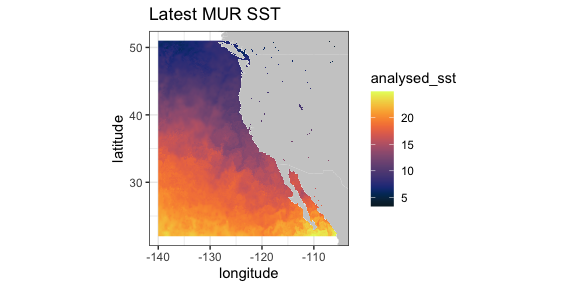### VIIRS SST and Chlorophyll

VIIRS (Visible Infrared Imaging Radiometer Suite) is a scanning radiometer, that collects visible and infrared imagery and radiometric measurements of the land, atmosphere, cryosphere, and oceans. VIIRS data is used to measure cloud and aerosol properties, ocean color, sea and land surface temperature, ice motion and temperature, fires, and Earth’s albedo. Both NASA and NOAA provide VIIRS-based high resolution SST and chlorophyll products.

ERD provides a 3-day composite SST product at 750 meter resolution developed from a real-time NOAA product. The most recent values can be obtained by setting “time” to be “last”. (Note that R sees the latitude-longitude grid as slightly uneven (even though it is in fact even), and that produces artificial lines in `ggplot2::geom_raster()`. In order to remove those lines, the latitude-longitude grid is remapped to an evenly-space grid.)

``````require("ggplot2")
require("mapdata")
require("rerddap")
sstInfo <- info('erdVHsstaWS3day')
# get latest 3-day composite sst
viirsSST <- griddap(sstInfo, latitude = c(41., 31.), longitude = c(-128., -115), time = c('last','last'), fields = 'sst')
# remap latitiudes and longitudes to even grid
myLats <- seq(range(myLats), range(myLats), length.out = length(myLats))
myLons <- seq(range(myLons), range(myLons), length.out = length(myLons))
# melt these out to full grid
mapFrame <- expand.grid(x = myLons, y = myLats)
mapFrame\$y <- rev(mapFrame\$y)
# form a frame with the new values and the data
tempFrame <- data.frame(sst = viirsSST\$data\$sst, lat = mapFrame\$y, lon = mapFrame\$x)
mycolor <- colors\$temperature
w <- map_data("worldHires", ylim = c(30., 42.), xlim = c(-128, -114))
ggplot(data = tempFrame, aes(x = lon, y = lat, fill = sst)) +
geom_polygon(data = w, aes(x = long, y = lat, group = group), fill = "grey80") +
geom_raster(interpolate = FALSE) +
scale_fill_gradientn(colours = mycolor, na.value = NA) +
theme_bw() + ylab("latitude") + xlab("longitude") +
coord_fixed(1.3, xlim = c(-128, -114),  ylim = c(30., 42.)) + ggtitle("Latest VIIRS 3-day SST")``````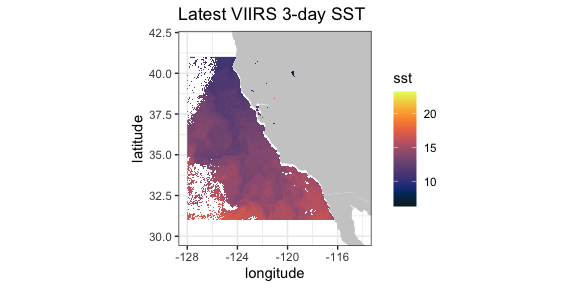A time series from the same dataset at a given location, here (36., -126.):

``````require("ggplot2")
require("rerddap")
viirsSST1 <- griddap(sstInfo, latitude = c(36., 36.),
longitude = c(-126., -126.),
time = c('2015-01-01','2015-12-31'), fields = 'sst')
tempTime <- as.Date(viirsSST1\$data\$time, origin = '1970-01-01', tz = "GMT")
tempFrame <- data.frame(time = tempTime, sst = viirsSST1\$data\$sst)``````
``````ggplot(tempFrame, aes(time, sst)) +
geom_line() +
theme_bw() +
ylab("sst") +
ggtitle("VIIRS SST at (36N, 126W)")``````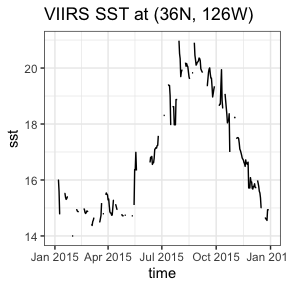A similar 3-day composite for chloropyll for the same region from a scientific quality product developed by NOAA:

``````require("ggplot2")
require("mapdata")
require("rerddap")
chlaInfo <- info('erdVHNchla3day')
viirsCHLA <- griddap(chlaInfo, latitude = c(41., 31.),
longitude = c(-128., -115), time = c('last','last'),
fields = 'chla')``````
``````mycolor <- colors\$chlorophyll
w <- map_data("worldHires", ylim = c(30., 42.), xlim = c(-128, -114))
ggplot(data = viirsCHLA\$data, aes(x = longitude, y = latitude, fill = log(chla))) +
geom_polygon(data = w, aes(x = long, y = lat, group = group), fill = "grey80") +
geom_raster(interpolate = FALSE) +
scale_fill_gradientn(colours = mycolor, na.value = NA) +
theme_bw() + ylab("latitude") + xlab("longitude") +
coord_fixed(1.3, xlim = c(-128, -114),  ylim = c(30., 42.)) +
ggtitle("Latest VIIRS 3-day Chla")``````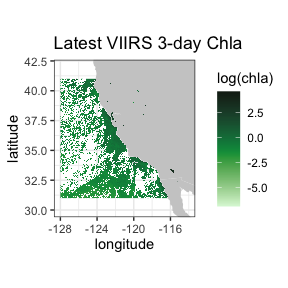### Temperature at 70m in the north Pacific from the SODA model output

This is an example of an extract from a 4-D dataset (results from the “Simple Ocean Data Assimilation (SODA)” model - see https://www2.atmos.umd.edu/~ocean/), and illustrate the case where the z-coordinate does not have the default name “altitude”. Water temperature at 70m depth is extracted for the North Pacific Ocean.

``````require("rerddap")
dataInfo <- rerddap::info('hawaii_d90f_20ee_c4cb')
xpos <- c(135.25, 240.25)
ypos <- c(20.25, 60.25)
zpos <- c(70.02, 70.02)
tpos <- c('2010-12-15', '2010-12-15')
soda70 <- griddap(dataInfo,  longitude = xpos, latitude = ypos,
time = tpos, depth = zpos, fields = 'temp' )``````

Since the data cross the dateline, it is necessary to use the new “world2Hires” continental outlines in the package `mapdata` which is Pacific Ocean centered. Unfortunatley there is a small problem where the outlines from certain countries wrap and mistakenly appear in plots, and those countries must be removed, see code below.

``````require("ggplot2")
require("mapdata")
xlim <- c(135, 240)
ylim <- c(20, 60)
my.col <- colors\$temperature
## Must do a kludge to remove countries that wrap and mess up the plot
w1 <- map("world2Hires", xlim = c(135, 240), ylim = c(20, 60), fill = TRUE, plot = FALSE)
remove <- c("UK:Great Britain", "France", "Spain", "Algeria", "Mali", "Burkina Faso", "Ghana", "Togo")
w <- map_data("world2Hires", regions = w1\$names[!(w1\$names %in% remove)], ylim = ylim, xlim = xlim)
myplot <- ggplot() +
geom_raster(data = soda70\$data, aes(x = longitude, y = latitude, fill = temp), interpolate = FALSE) +
geom_polygon(data = w, aes(x = long, y = lat, group = group), fill = "grey80") +
theme_bw() + scale_fill_gradientn(colours = my.col, na.value = NA, limits = c(-3,30), name = "temperature") +
ylab("latitude") + xlab("longitude") +
coord_fixed(1.3, xlim = xlim, ylim = ylim) +
ggtitle(paste("70m temperature ", soda70\$data\$time))
myplot``````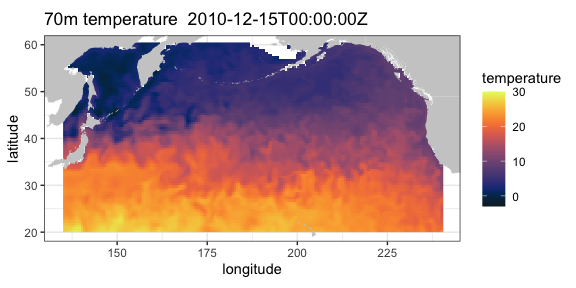### Irish Marine Institute

The Irish Marine Institute has an ERDDAP server at https://erddap.marine.ie/erddap. Among other datasets, they have hourly output from a model of the North Altantic ocean, with a variety of ocean related parameters, see the dataset IMI_NEATL. To obtain the latest sea surface salinity for the domain of the model:

``````require("rerddap")
urlBase <- "https://erddap.marine.ie/erddap/"
parameter <- "sea_surface_salinity"
sssTimes <- c("last", "last")
sssLats <- c(48.00625, 57.50625)
sssLons <- c(-17.99375, -1.00625)
dataInfo <- rerddap::info("IMI_NEATL", url = urlBase)
NAtlSSS <- griddap(dataInfo, longitude = sssLons, latitude = sssLats, time = sssTimes, fields = parameter, url = urlBase)``````

and the extracted data plotted:

``````require("ggplot2")
require("mapdata")
xlim <- c(-17.99375, -1.00625)
ylim <- c(48.00625, 57.50625)
my.col <- colors\$salinity
w <- map_data("worldHires", ylim = ylim, xlim = xlim)
myplot <- ggplot() +
geom_raster(data = NAtlSSS\$data, aes(x = longitude, y = latitude, fill = sea_surface_salinity), interpolate = FALSE) +
geom_polygon(data = w, aes(x = long, y = lat, group = group), fill = "grey80") +
theme_bw() + scale_fill_gradientn(colours = my.col, na.value = NA, limits = c(34, 36), name = "salinity") +
ylab("latitude") + xlab("longitude") +
coord_fixed(1.3, xlim = xlim, ylim = ylim) +
ggtitle(paste("salinity", NAtlSSS\$data\$time))
myplot``````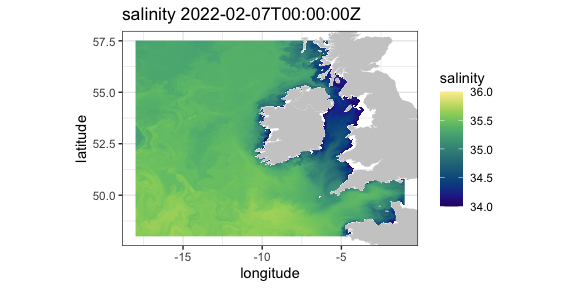### IFREMER

The French agency IFREMER also has an ERDDAP server. Here salinity data at 75 meters from “Global Ocean, Coriolis Observation Re-Analysis CORA4.1” model off the west coast of the United States is extracted and plotted.

``````require("rerddap")
urlBase <- "https://www.ifremer.fr/erddap/"
parameter <- "PSAL"
ifrTimes <- c("2019-05-15", "2019-05-15")
ifrLats <- c(30., 50.)
ifrLons <- c(-140., -110.)
ifrDepth <- c(75., 75.)
dataInfo <- rerddap::info("CORIOLIS_GLOBAL_NRTOA_OBS_TIME_SERIE_PSAL", url = urlBase)
ifrPSAL <- griddap(dataInfo, longitude = ifrLons, latitude = ifrLats, time = ifrTimes, depth = ifrDepth,  fields = parameter, url = urlBase)``````

The `ggplot2` function `geom_raster()` is not designed for unevenly spaced coordinates, as are the latitudes from this model. The function `interp()` from the package `akima` is used to interpolate the data which are then plotted.

``````## ggplot2 has trouble with unequal y's
require("akima")
require("dplyr")
require("ggplot2")
require("mapdata")
xlim <- c(-140, -110)
ylim <- c(30, 51)
## ggplot2 has trouble with unequal y's
my.col <- colors\$salinity
tempData1 <- ifrPSAL\$data\$PSAL
tempData <- array(tempData1 , 61 * 54)
tempFrame <- data.frame(x = ifrPSAL\$data\$longitude, y = ifrPSAL\$data\$latitude)
tempFrame\$temp <- tempData
tempFrame1 <- dplyr::filter(tempFrame, !is.nan(temp))
myinterp <- akima::interp(tempFrame1\$x, tempFrame1\$y, tempFrame1\$temp, xo = seq(min(tempFrame1\$x), max(tempFrame1\$x), length = 61), yo = seq(min(tempFrame1\$y), max(tempFrame1\$y), length = 54))
myinterp1 <- expand.grid(x = myinterp\$x, y = myinterp\$y)
myinterp1\$temp <- array(myinterp\$z, 61 * 54)
w <- map_data("worldHires", ylim = ylim, xlim = xlim)
myplot <- ggplot() +
geom_raster(data = myinterp1, aes(x = x, y = y, fill = temp), interpolate = FALSE) +
geom_polygon(data = w, aes(x = long, y = lat, group = group), fill = "grey80") +
theme_bw() + scale_fill_gradientn(colours = my.col, na.value = NA, limits = c(32, 35), name = "salinity") +
ylab("latitude") + xlab("longitude") +
coord_fixed(1.3, xlim = xlim, ylim = ylim) + ggtitle(paste("salinity at 75 meters",ifrPSAL\$data\$time ))
myplot``````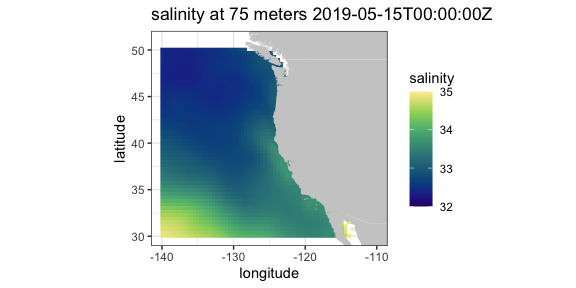## tabledap

### CalCOFI data

CalCOFI (California Cooperative Oceanic Fisheries Investigations - https://calcofi.org) is a multi-agency partnership formed in 1949 to investigate the collapse of the sardine population off California. The organization’s members are from NOAA Fisheries Service, Scripps Institution of Oceanography, and California Department of Fish and Wildlife. The scope of this research has evolved into the study of marine ecosystems off California and the management of its fisheries resources. The nearly complete CalCOFI data, both physical and biological, are available through ERDDAP.

The following example is a modification of a script developed by Dr. Andrew Leising of the Southwest Fisheries Science Center. The original script has been used to automate the generation of several yearly reports about the California Current Ecosystem. The script gets chlorophyll data and a measure of primary productivity from the hydrocasts,and then calculates a seasoanlly adjusted chlorophyll anomaly as well as a seasonally adjusted primary productivity anomaly. The first step is to get the information about the particular dataset:

``````require("rerddap")
hydroInfo <- info('siocalcofiHydroCast')``````

and then get the desired data from 1984 through 2014:

``````require("rerddap")
calcofi.df <- tabledap(hydroInfo, fields = c('cst_cnt',  'date', 'year', 'month', 'julian_date', 'julian_day', 'rpt_line', 'rpt_sta', 'cruz_num', 'intchl', 'intc14', 'time'), 'time>=1984-01-01T00:00:00Z', 'time<=2014-04-17T05:35:00Z')``````

Both “intchl” and “intC14” are returned as character strings, and are easier to work with as numbers:

``````calcofi.df\$cruz_num <- as.numeric(calcofi.df\$cruz_num)
calcofi.df\$intc14 <- as.numeric(calcofi.df\$intc14)
calcofi.df\$time <- as.Date(calcofi.df\$time, origin = '1970-01-01', tz = "GMT")``````

At this point the requested data are in the R workspace - the rest of the code performs the calculations to derive the seasonally adjusted values and plot them.

``````require("dplyr")

# calculate cruise means
by_cruznum <- group_by(calcofi.df, cruz_num)
tempData <- select(by_cruznum, year, month, cruz_num, intchl, intc14)
CruiseMeans <- summarize(by_cruznum, cruisechl = mean(intchl, na.rm = TRUE), cruisepp = mean(intc14, na.rm = TRUE), year = median(year, na.rm = TRUE), month = median(month, na.rm = TRUE))
tempTimes <- paste0(CruiseMeans\$year,'-',CruiseMeans\$month,'-1')
cruisetimes <- as.Date(tempTimes, origin = '1970-01-01', tz = "GMT")
CruiseMeans\$cruisetimes <- cruisetimes
# calculate monthly "climatologies"
byMonth <- group_by(CruiseMeans, month)
climate <- summarize(byMonth, ppClimate = mean(cruisepp, na.rm = TRUE), chlaClimate = mean(cruisechl, na.rm = TRUE))
# calculate anomalies
CruiseMeans\$chlanom <- CruiseMeans\$cruisechl - climate\$chlaClimate[CruiseMeans\$month]
CruiseMeans\$ppanom <- CruiseMeans\$cruisepp - climate\$ppClimate[CruiseMeans\$month]
# calculate mean yearly anomaly
byYear <- select(CruiseMeans, year)
tempData <- select(CruiseMeans, year, chlanom, ppanom )
byYear <- group_by(tempData, year)
yearlyAnom <- summarize(byYear, ppYrAnom = mean(ppanom, na.rm = TRUE), chlYrAnom = mean(chlanom, na.rm = TRUE))
yearlyAnom\$year <- ISOdate(yearlyAnom\$year, 01, 01, hour = 0)
ggplot(yearlyAnom, aes(year, chlYrAnom)) + geom_line() +
theme_bw() + ggtitle('yearly chla anom')
ggplot(yearlyAnom, aes(year, ppYrAnom)) + geom_line() +
theme_bw() + ggtitle('yearly pp anom')``````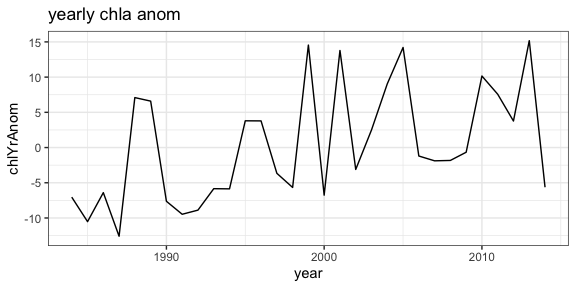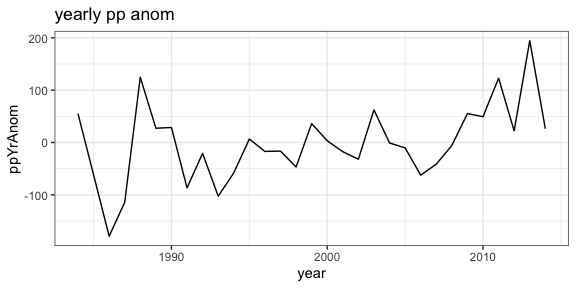### CPS Trawl Surveys

The CPS (Coastal Pelagic Species) Trawl Life History Length Frequency Data contains the length distribution of a subset of individuals from a species (mainly non-target) caught during SWFSC-FRD fishery independent trawl surveys of coastal pelagic species. Measured lengths for indicated length type (fork, standard, total, or mantle) were grouped in 10 mm bins (identified by the midpoint of the length class) and counts are recorded by sex.

The number and location of sardines (Sardinops sagax) in the tows in March 2010 and 2011 are extracted, and compared with monthly SST from satellites. First, query the ERDDAP server to see if CPS Trawl data are available through the ERDDAP server, and if so, obtain the datasetID for the dataset.

``````require("rerddap")
CPSquery <- ed_search(query = 'CPS Trawl')
CPSquery\$alldata[]\$summary
CPSquery\$alldata[]\$tabledap
CPSquery\$alldata[]\$dataset_id``````

Then get the information for the CPS dataset:

``````require("rerddap")
CPSinfo <- info('FRDCPSTrawlLHHaulCatch')``````

extract the desired CPS data:

``````require("dplyr")
require("rerddap")
sardines <- tabledap(CPSinfo, fields = c('latitude',  'longitude', 'time', 'scientific_name', 'subsample_count'), 'time>=2010-01-01', 'time<=2012-01-01', 'scientific_name="Sardinops sagax"' )
sardines\$time <- as.Date(sardines\$time, origin = '1970-01-01', tz = "GMT")
sardines\$latitude <- as.numeric(sardines\$latitude)
sardines\$longitude <- as.numeric(sardines\$longitude)
sardine2010 <- filter(sardines, time < as.Date('2010-12-01'))``````

and plot the data versus monthly SST values:

``````# get the dataset info
sstInfo <- info('erdMWsstdmday')
# get 201004 monthly sst
sst201004 <- griddap('erdMWsstdmday', latitude = c(22., 51.), longitude = c(220., 255), time = c('2010-04-16','2010-04-16'), fields = 'sst')
# get 201104 monthly sst
sst201104 <- griddap('erdMWsstdmday', latitude = c(22., 51.), longitude = c(220., 255), time = c('2011-04-16','2011-04-16'), fields = 'sst')``````
``````# get polygons of coast for this area
w <- map_data("worldHires", ylim = c(22., 51.), xlim = c(220 - 360, 250 - 360))
# plot 201004 sst on the map
sardine2010 <- filter(sardines, time < as.Date('2010-12-01', origin = '1970-01-01', tz = "GMT"))
sardine2011 <- filter(sardines, time > as.Date('2010-12-01', origin = '1970-01-01', tz = "GMT"))
mycolor <- colors\$temperature
p1 <- ggplot() +
geom_polygon(data = w, aes(x = long, y = lat, group = group), fill = "grey80") +
geom_raster(data = sst201004\$data, aes(x = (longitude - 360), y = latitude, fill = sst), interpolate = FALSE) +
scale_fill_gradientn(colours = mycolor, na.value = NA, limits = c(5,30)) +
theme_bw() + ylab("latitude") + xlab("longitude") +
coord_fixed(1.3, xlim = c(220 - 360, 250 - 360),  ylim = c(22., 51.))

# plot 201104 sst on the map
p2 <- ggplot() +
geom_polygon(data = w, aes(x = long, y = lat, group = group), fill = "grey80") +
geom_raster(data = sst201104\$data, aes(x = (longitude - 360), y = latitude, fill = sst), interpolate = FALSE) +
geom_point(data = sardine2011, aes(x = longitude, y = latitude, colour = subsample_count)) +
scale_fill_gradientn(colours = mycolor, na.value = NA, limits = c(5,30)) +
theme_bw() + ylab("latitude") + xlab("longitude") +
coord_fixed(1.3, xlim = c(220 - 360, 250 - 360),  ylim = c(22., 51.))
p1 + geom_point(data = sardine2010, aes(x = longitude, y = latitude, colour = subsample_count)) + scale_colour_gradient(space = "Lab", na.value = NA, limits = c(0,80))

p2 +   geom_point(data = sardine2011, aes(x = longitude, y = latitude, colour = subsample_count)) + scale_colour_gradient(space = "Lab", na.value = NA, limits = c(0,80))``````